# ETAA Final Math Part Deux

#### Numerical Sequences

For Questions #1-#4, find the missing number in each numeric sequence.

#### Plug In PEMDAS

For Questions #5-#6, substitute the numbers for the variables, simplify, and find the numeric solution.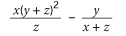For Questions #7-#8, substitute the numbers for the variables, simplify, and find the numeric solution.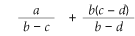For Questions #9-#10, substitute the numbers for the variables, simplify, and find the numeric solution.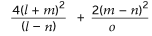For Questions #11-#12, substitute the numbers for the variables, simplify, and find the numeric solution.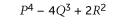For Questions #13-#14, substitute the numbers for the variables, simplify, and find the numeric solution.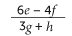For Questions #15-#16, substitute the numbers for the variables, simplify, and find the numeric solution.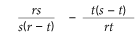#### Linear Equations

For Questions #17-#22, Match the Linear Equation expression in the question to the identical linear equation in the answers.
17.A. thirty-five more than twice a number
B. y = –2x + 32
C.D. forty more than the opposite of a number

ABCD

18.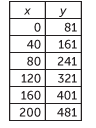A. Eighty-one more than a number
B. y = 2x + 81
C. Eighty-one less than three times a number
D.ABCD

19. y = 2x + 20
A.B. Twenty more than the opposite of a number
C.D. twenty more than a number

ABCD

20. One hundred and twenty three more than twice a number
A.B. y = x + 123
C.D. y = 2x + 123

ABCD

21.A. y = 2x + 71
B.C. y = x + 29
D. thirty more than the opposite of a number

ABCD

22. Twenty more than a number

A. y = –x + 20
B.C.D.ABCD

#### Dimensional Analysis

For Questions #23-#27, apply the relationships between the variables of the equation to determine the correct answer.

23. R = STV

In the formula above, if R is negative and S is positive, which of the following statements could be true?

A. T and V are both positive.
B. T and V are both negative.
C. R, S and T are all negative.
D. T is negative and V is positive.

ABCD

24. f =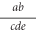In the formula above, if f increases and a, c, and d all remain constant, which of the following statements could be true?

A. e remains constant and b increases.
B. b remains constant and e increases.
C. e remains constant and b decreases.
D. b and e both remain constant.

ABCD

25. y = 3 – 5x

Which of the following statements is true for the formula above?

A. If x > 3, then y > 0.
B. If x = 0, then y < 0.
C. If x > 3/5, then y < 0.
D. If x < 3/5, then y < 0.

ABCD

26. d =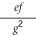In the formula above, if e, f, and g are all the same sign, which of the following statements must be true?

A. d and e are both negative.
B. f is positive and d is negative.
C. d and f are both negative.
D. d and e are both positive.

ABCD

27. h = 4dWhich of the following statements must be true for the formula above?

A. If d < 1/4 and f < 7, then h is positive.
B. If d > 1/4 and f < 7, then h is positive.
C. If d < 0 and f = 7, then h is positive.
D. If d > 1 and f > 7, then h is negative.

ABCD

#### Variable Flip

For Questions #28-#32, isolate the variable on the right side of the equation.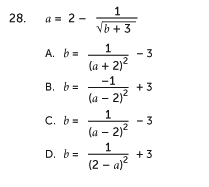ABCD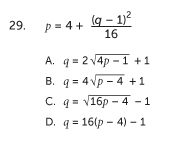ABCD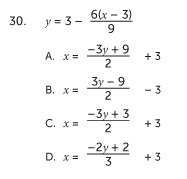ABCD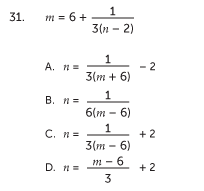ABCD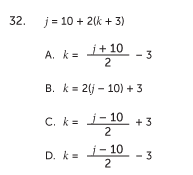ABCD

##### STOP

Unauthorized copying or reuse of any part of this page is illegal.